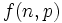# Higman-Sims asymptotic formula on number of groups of prime power order

## Statement

### Quick version

Let$p$ be a (fixed) prime number. Define$f(n,p)$ as the number of groups of order$p^n$ (see also group of prime power order, number of groups of given order). Then:$f(n,p) = p^{(2/27 + o(1))n^3}$

### Full version

Let$p$ be a (fixed) prime number. Define$f(n,p)$ as the number of groups of order$p^n$ (see also group of prime power order, number of groups of given order). Define:$A(n,p) := \frac{\log f(n,p)}{n^3 \log p}$

Then:$A(n,p) = \frac{2}{27} + O(n^{-1/3})$

Note that the big-O notation is with respect to$n$ (sending$n \to \infty$ and not with respect to$p$). However, the constants under the big-O notation may depend on$p$.